output.to from Sideway
Mechanics Dynamics

Kinematics

Kinematics

Draft for Information Only

# Content

``` Mechanics: Kinematics   Position   Distance   Displacement   Speed   Velocity   Acceleration   Idealizations```

## Mechanics: Kinematics

Kinematics is the study of the geometry of an object's motion which is related to the position, velocity, and acceleration with respect to time.

### Position

The position of an object is used to specify where the object is when describing the motion of the object. Although position is used to describe the location of an object in daily life, a position is usually considered as a vector in mechanics and physics because a position is always expressed in term of a magnitude and a direction with reference to a reference point or a zero point, that is the origin point. For example, origin O, position A, position B, and position C.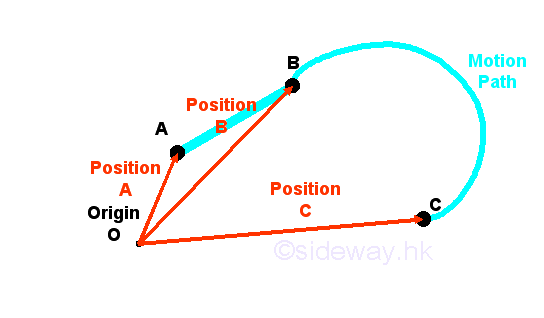### Distance

The term distance is not a vector because a distance is only the measured magnitude between two positions with reference to each other. For example, distance between position A and position B, distance between position B and position C, and distance between position C and position A. Although distance is defined the shortest length of two positions, but travelled distance is used to described the path of the motion of the object. Therefore the total travelled distance from A to B to C is equal to the sum of path between position A and position B and path between position B and position C.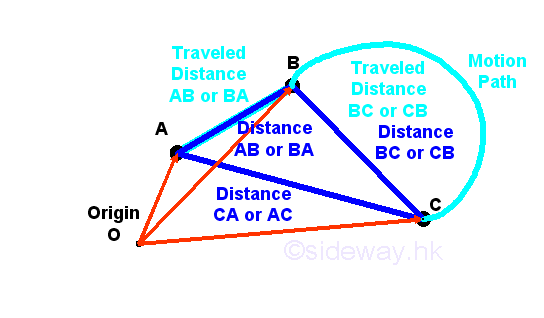### Displacement

But, the term displacement is a vector used to specify the motion of an object, which has a magnitude and a direction also,  from one position to another position.  For example, displacement form position A to position C. Unlike the term distance, the term displacement usually refers to the shortest motion from the start position to the end position only.### Speed

Besides where an object is or where an object is going to, how fast the object is moving is also important when describing the motion of an obeject. The generic term speed is a magnitude used to describe how fast is the object moving. Although, the term speed is usually refered to the instantaneous speed of the motion of an object, sometimes, speed is also used to describe the average speed of the motion of an object. An instantaneous speed of the motion of an object is the speed of the motion of an object at a particular instant in time or at a particular instant in position. Average speed is defined as the total travelled distance over the time interval of the time travelled. Since speed is a scalar, average speed is therefore also equal to the average of the instantaneous speed of the motion of an object within a time interval or over the total distance covered.  In other words, instantaneous speed at a particular instant in time is equal to the average speed of the motion of an object when the time travelled interval is sufficiently small such that the speed over that time interval can be considered to be a constant.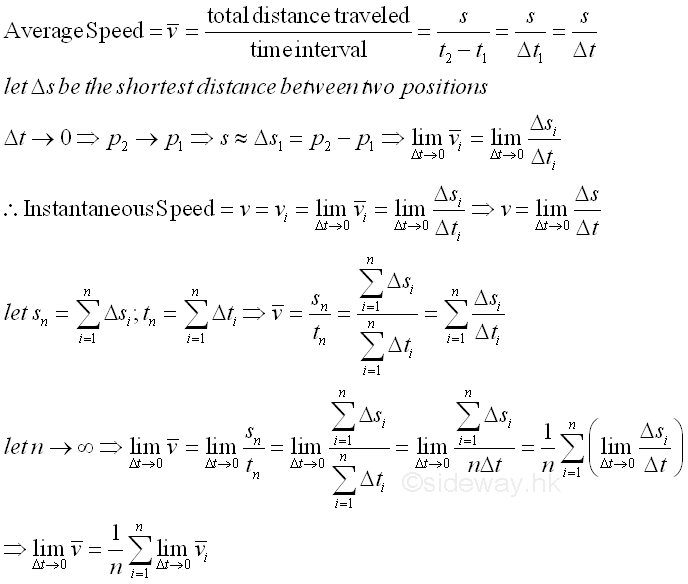### Velocity

Although the meaning of the term velocity is often interchangeable with the term speed in daily life, however velocity and speed are different in mechanics or physics. Velocity always has both a direction and a magnitude while speed has a magnitude only. In other words, velocity is a vector and therefore unlike speed, the direction of the motion of an object should be considered when determining the velocity of the object. Similar to average speed, average velocity is defined as the displacement of an object over the time interval of the time travelled from the start position to the end position. Since velocity is a vector quantity with direction from start position to end position that is equal to end position vector minus start position vector. The magnetude of average velocity is therefore equal to the average rate of the change of position or the net displacement with respect to time. And the direction of the average velocity is same as the direction of the displacement. And therefore average velocity of an object is not always equal to the average speed of an object.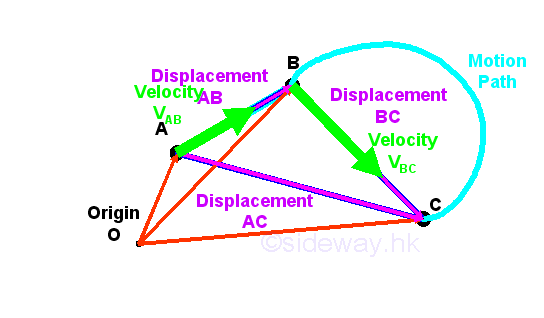Similarly, for a vector quantity, the average velocity vector is equal to the average of the vector sum of the instantaneous velocity of the motion of displacement of an object within a time interval or over the displacement covered. And the definition of instantaneous velocity is also equal to the vector representation of the rate of change of position vector with respect to time at a particular instant in time when the travelled time interval is sufficiently small such that the velocity vector over that time interval can be considered to be a constant and the travelled distance over that time interval can be considered to be equal to a linear displacement.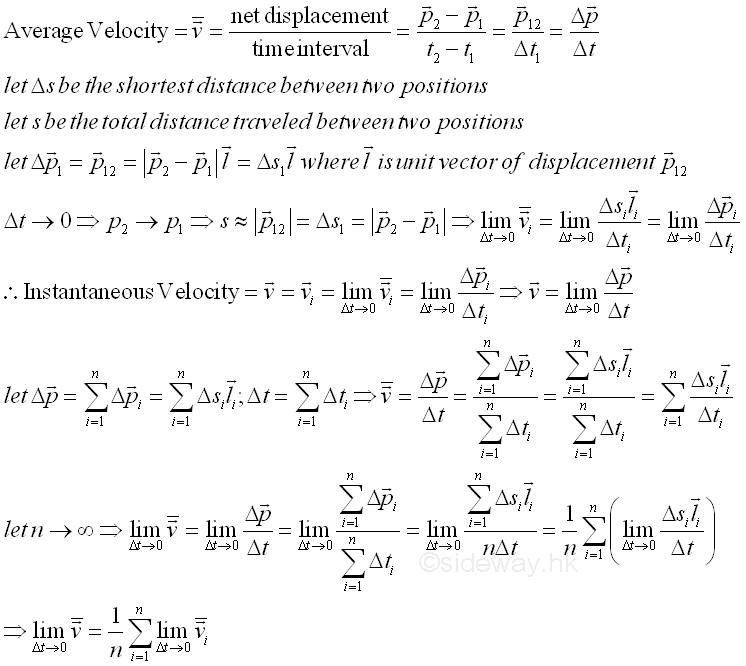### Acceleration

In daily life, acceleration is a generic term used to describe how the speed of the motion of an object changes with time . But in mechanics or physics, acceleration is a vector quantity and is defined as the rate of an object changes its velocity from a position to another position over a time interval. In general, an object is accelerating if either the speed or velocity of the object changes with time. The acceleration of the speed of an object is a descriptive scalar quantity which is usually used to describe the movement of an object, e.g. how fast to speed up, to slow down, or to turn. While the acceleration of the velocity of an object is a vector quantity to represent the rate of net change of velocities at the start and end positions. Since acceleration is a vector quantity with direction equals to end velocity vector minus start velocity vector. The magnetude of average acceleration is therefore equal to the average rate of the change of velocity with respect to time.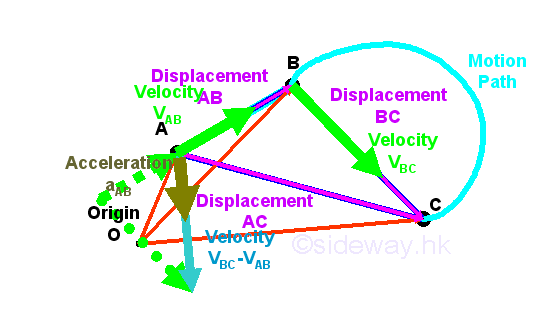Similarly, for a vector quantity, the average acceleration vector is equal to the average of the vector sum of the instantaneous acceleration of the motion of displacement of an object within a time interval or over the displacement covered. And the definition of instantaneous acceleration is also equal to the vector representation of the rate of change of velocity vector with respect to time at a particular instant in time when the travelled time interval is sufficiently small such that the acceleration vector over that time interval can be considered to be a constant, and the rate of change of the velocity over that time interval can be considered to be linear.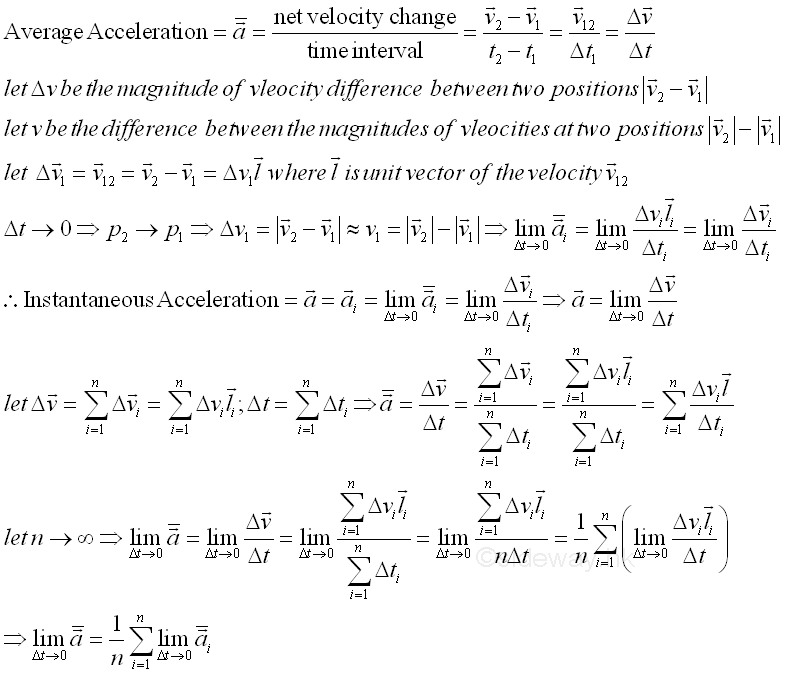### Idealizations

Idealizations are techniques used to simplify the application of theory in problem modeling. They arem modeling. They are

• Particle is a point mass and its size can be neglected

• Rigid Body is one single object formed by a combination of particles in which all particles remain at a fixed distance from one another and are assumed to be rigid under applied forces.

• Concentrated Force is a mathematical representation of the effect of forces acting on a rigid body by assuming a single force acts at a point on the object along a single line of force in space.

©sidewayReferences

1. I.C. Jong; B.G. rogers, 1991, Engineering Mechanics: Statics and Dynamics, Saunders College Publishing, United States of America
2. F.P. Beer; E.R. Johnston,Jr.; E.R. Eisenberg, 2004, Vector Mechanics for Engineers: Statics, McGraw-Hill Companies, Inc., New YorkID: 140200001 Last Updated: 2014/2/1 Revision: 0 Ref:Home (5)

Business

Management

HBR (3)

Information

Recreation

Hobbies (7)

Culture

Chinese (1097)

English (336)

Reference (66)

Computer

Hardware (149)

Software

Application (187)

Digitization (24)

Numeric (19)

Programming

Web (644)CSS (SC)

ASP.NET (SC)

HTML

Knowledge Base

Common Color (SC)

Html 401 Special (SC)

OS (389)MS Windows

Windows10 (SC)

.NET Framework (SC)

DeskTop (7)

Knowledge

Mathematics

Formulas (8)

Number Theory (206)

Algebra (20)

Trigonometry (18)

Geometry (18)

Calculus (67)

Complex Analysis (21)

Engineering

Tables (8)

Mechanical

Mechanics (1)

Rigid Bodies

Statics (92)

Dynamics (37)

Fluid (5)

Control

Acoustics (19)

Biology (1)

Geography (1)

Latest Updated Links

Copyright © 2000-2019 Sideway . All rights reserved Disclaimers last modified on 10 Feb 2019